# Ex: Multiplying Using the Distributive Property

##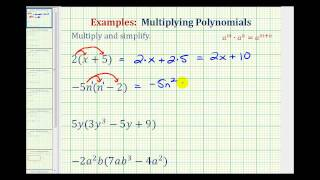By Mathispower4u

This video provides examples of multiplication of a monomial and a binomial as well as a monomial and a trinomial using the distributive property.

# Binomial Expansion Using Pascal's Triangle

##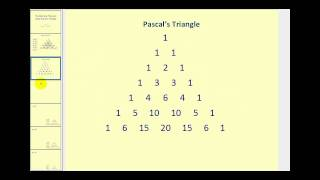By Mathispower4u

This video explains binomial expansion using Pascal's triangle.

# Division of Rational Expressions

##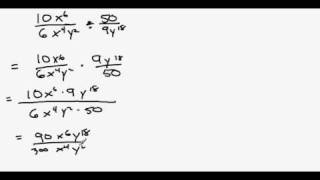By MrLovellFord

Shows how to divide two rational expressions by mulitplying the first expression by the reciprocal of the second, then simplifying.

# Arithmetic Series

##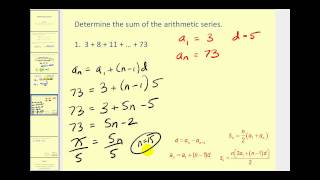By Mathispower4u

Arithmetic Series

# Finding the Inverse of a Function or Showing One Does not Exist, Ex 3

##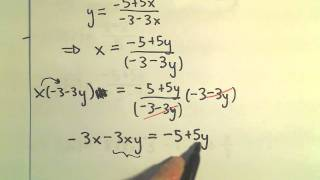By PatrickJMT

YouTube presents Finding the Inverse of a Function or Showing One Does not Exist, Ex 3, an educational video resource on math.

# 1.8 How to find the inverse of a linear function- Online Tutor- Free Math Videos

##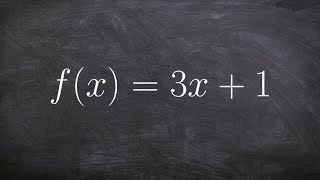By Mr. McLogan's Math Channel

In this video tutorial I will show you how to find the inverse of a function f(x). I do this by first writing the equation substituting y for f(x). I then solve the equation for x. The result is the inverse. The inverse can be written, substituting y back in for x, and x for y. I also show how to verify that a function is an inverse of another function.I show how to solve math problems online during live instruction in class. This is my way of providing free tutoring for the students in my class and for students anywhere in the world. Every video is a short clip that shows exaclty how to solve math problems step by step. The problems are done in real time and in front of a regular classroom. These videos are intended to help you learn how to solve math problems, review how to solve a math problems, study for a test, or finish your homework.I post all of my videos on youtube but if you are looking for other ways to interact with me and my videos you ca

# Graphing a cosine function in form y=AcosB(x-C)+D --- EASY!!!!

##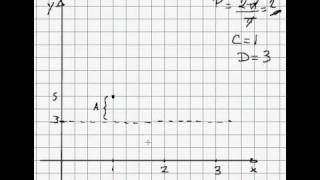By AcademicLeadersEd

Graphing a cosine function while interpreting the values of A, B, C and D, yielding amplitude, period, phase shift and vertical displacement of the cosine graph.

# cosine and sine values on the unit circle

##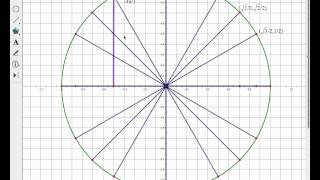By David Metzler

Two quick, basic problems about cosine and sine values on the unit circle

# 10.6 More Angle-Arc Theorems (Practice)

##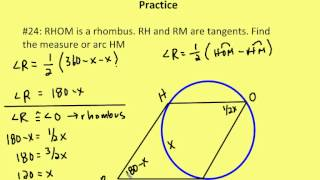By AutenMath

A lesson on 1.) the relationship of inscribed and tangent-chord angles with the same or congruent arcs 2.) the measure of an inscribed angle whose intercepted arc is a semicircle and 3.) the relationship of tangent-tangent angles and their minor arcs

# Part 2 of proof of Heron's formula | Perimeter, area, and volume | Geometry | Khan Academy

##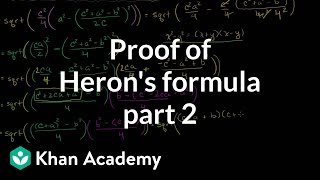By Khan Academy

Video shows that the expression in part 1 is identical to Heron's Formula. This video demonstrates how to use Heron's Formula to determine the area of a triangle while only knowing the lengths of the sides

...